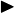# Rectifier Linear Unit (ReLU)

Rectifier Linear Unit (ReLU), which was first introduced by Nair and Hinton in 2010, is arguably the most important and frequently used activation function in this age of Deep Learning thus far.

Despise its simplicity, ReLU previously achieved the top performance over various tasks of modern Machine Learning, including but not limited to Nature Language Processing (NLP), Voice Synthesis and Computer Vision (CV). This blog post attempts to give an introduction to this honored function and elaborate on the reasons why it works so well.

The formula of ReLU is simply:

f(x) = max(0, x)

which means a positive value is kept as it is while 0 is outputted if the input is less than or equal to 0.

Compared to traditional candidates like Sigmoid or Tanh, the output of ReLU is not bounded in a finite range. In other words, ReLU is a non-saturated nonlinearity.

While the exact causes of ReLU’s success are still not fully clear, in this blog, we present the common consensus and theoretical reasoning about its advantages and weaknesses.Fast. The ReLU activation consists of just a thresholding operation, which is more speedy than the expensive exponential calculation in Sigmoid and Tanh.More robust against Vanishing Gradient problem. Both Sigmoid and Tanh have their absolute derivative value small, which makes the gradient gradually vanish at each and every layer when flowing backward. With ReLU, if the input value is larger than 0, the derivative is 1, helping the gradient to keep its strength when propagating.It reserves the nature of gradient in backpropagation. The derivative of ReLU is either 1 (for positive inputs) or 0, which, respectively, leads to 2 options: to keep the gradients flow back as it is or do not let it get through at all.

The learning gradients normally involve multiplying many derivatives and weights together as they flow backward. Over these, the weights are intuitively more important, since the derivatives, as they depend on the type of activation function, are more of tuning parameters with the initial intent of introducing nonlinearity to the networks. Hence, it makes sense to limit the effect of activation functions in the process of calculating gradient size.Sparsity. As it thresholds at 0, many of the signals coming to the ReLU activation will then be eliminated, which makes the model more sparse than with traditional functions. Sparsity has its own good points:

• It is less sensitive to small changes in the input. A robust model should not be over-influenced by some small changes in the input values. By being sparse, many energy flows in the networks will be blocked at some point (at some neuron), making them not able to affect the result of the networks. Furthermore, for example, a noisy small variant of the input data changes the input of some neurons, from -11 to -10, this has no effects at all because the output is 0 indifferently. Notice that these small changes include not only noise but also, for example, the relocation of subjects in image data.
• Dynamic network size. As various input requires various network sizes (which are determined by hyper-parameters like the number of neurons in each layer), sparsity somehow relieves us of this burden to find the optimum hyper-parameters. The best number of active neurons can be learned from the input itself.
• It is often easier for classifiers to work in high-and-sparse dimensional space than moderate-and-dense space.

WeaknessesNon-zero centered. ReLU suffers the same problem as Sigmoid, while their values are wrapped to the non-negative region (actually, outputs of Sigmoid function are all positive). This property forces all weights connecting to a neuron to move the same direction (to be added or to be subtracted by a positive amount) in the backpropagation process.ReLU suffers from Exploding Gradient problem (while traditional Sigmoid and Tanh do not). The output of ReLU is unbounded, which means it can be very large, and when we multiply many large numbers together, the result is exponentially exploding. In practice, there are techniques to handle this problem, like normalization (BatchNorm, for instance) or Gradient clipping (e.g. clip gradients by a global norm of 5.0).The dying ReLU problem. As it thresholds at 0, many inputs flow into a neuron and “die” there (the inputs with non-positive values). As the activation outputs 0, the gradient is also 0, which means it cannot learn anything from the backward-learning phase and remains inactive eternally. While this can potentially have some good effects (as we discussed in the above section), some argue that it is detrimental to the networks. There are many variants of ReLU that appear to resolve this problem, including Leaky ReLU, PReLU, and ELU.

Note that the dying ReLU is also a type of Vanishing Gradient Problem.In practice, ReLU is more likely to overfit. Thus, more data is required, regularization techniques (e.g. dropout) are also beneficial.

Conclusion

ReLU and its siblings are indeed an indispensable part of the current state of Deep Learning. However, the success of ReLU did not come by itself alone, but in combination with other renowned techniques. For example, BatchNorm helps deal with ReLU’s problem of Exploding Gradient Problem, Dropout helps prevent over-fitting.

In the next blog posts, we will visit the other members of ReLU’s family to see how they address ReLU’s problems.

References:

1.Sean O'Connor says: